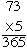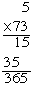Name: Marty elementary paraeducator This is a curiosity question. When multiplying do you put the number you are multiplying by on the top or the bottom. Example if you are multiplying by 6's would you write the problem    6 x 2 or    2 x 6 Maybe it just doesn't matter. But when you get to double digits, the double digit always goes on the top? Hi Marty, Since 2 times 6 and 6 times 2 are both 12, mathematically it doesn't matter which order you use. However if you asked me to multiply five by six I would probably write 5 x 6 or    5 x 6 since that's the order of the words five and six in the phrase "multiply five by six". Likewise for multiplying a two digit number and a one digit number, the product is the same regardless of the order. The proceduregives the same result asHere I would choose to write the two digit number above the one digit number as carrying out the procedure is easier than with the one digit number on top. Cheers, Penny Go to Math Central